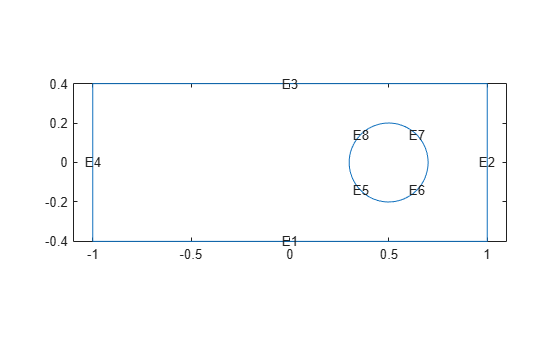# Solve PDEs with Constant Boundary Conditions

This example shows how to apply various constant boundary condition specifications for both scalar PDEs and systems of PDEs.

### Geometry

All the specifications use the same 2-D geometry, which is a rectangle with a circular hole.

```% Rectangle is code 3, 4 sides, followed by x-coordinates and then y-coordinates R1 = [3,4,-1,1,1,-1,-.4,-.4,.4,.4]'; % Circle is code 1, center (.5,0), radius .2 C1 = [1,.5,0,.2]'; % Pad C1 with zeros to enable concatenation with R1 C1 = [C1;zeros(length(R1)-length(C1),1)]; geom = [R1,C1]; % Names for the two geometric objects ns = (char('R1','C1'))'; % Set formula sf = 'R1 - C1'; % Create geometry g = decsg(geom,sf,ns); % Create geometry model model = createpde; % Include the geometry in the model and view the geometry geometryFromEdges(model,g); pdegplot(model,'EdgeLabels','on') xlim([-1.1 1.1]) axis equal```### Scalar Problem

Suppose that edge 3 has Dirichlet conditions with value 32, edge 1 has Dirichlet conditions with value 72, and all other edges have Neumann boundary conditions with `q = 0`, `g = -1`.

```applyBoundaryCondition(model,'dirichlet','edge',3,'u',32); applyBoundaryCondition(model,'dirichlet','edge',1,'u',72); applyBoundaryCondition(model,'neumann','edge',[2,4:8],'g',-1);```

This completes the boundary condition specification.

Solve an elliptic PDE with these boundary conditions with `c = 1`, `a = 0`, and `f = 10`. Because the shorter rectangular side has length 0.8, to ensure that the mesh is not too coarse choose a maximum mesh size `Hmax = 0.1`.

```specifyCoefficients(model,'m',0,'d',0,'c',1,'a',0,'f',10); generateMesh(model,'Hmax',0.1); results = solvepde(model); u = results.NodalSolution; pdeplot(model,'XYData',u,'ZData',u) view(-23,8)```### System of PDEs

Suppose that the system has `N = 2`.

• Edge 3 has Dirichlet conditions with values [32,72].

• Edge 1 has Dirichlet conditions with values [72,32].

• Edge 4 has a Dirichlet condition for the first component with value 52, and has a Neumann condition for the second component with `q = 0`, `g = -1`.

• Edge 2 has Neumann boundary conditions with `q = [1,2;3,4]` and `g = [5,-6]`.

• The circular edges (edges 5 through 8) have `q = 0` and `g = 0`.

```model = createpde(2); geometryFromEdges(model,g); applyBoundaryCondition(model,'dirichlet','edge',3,'u',[32,72]); applyBoundaryCondition(model,'dirichlet','edge',1,'u',[72,32]); applyBoundaryCondition(model,'mixed','edge',4,'u',52,'EquationIndex',1,'g',[0,-1]); Q2 = [1,2;3,4]; G2 = [5,-6]; applyBoundaryCondition(model,'neumann','edge',2,'q',Q2,'g',G2); % The next step is optional, because it sets 'g' to its default value applyBoundaryCondition(model,'neumann','edge',5:8,'g',[0,0]);```

This completes the boundary condition specification.

Solve an elliptic PDE with these boundary conditions using `c = 1`, `a = 0`, and `f = [10;-10]`. Because the shorter rectangular side has length 0.8, to ensure that the mesh is not too coarse choose a maximum mesh size `Hmax = 0.1`.

```specifyCoefficients(model,'m',0,'d',0,'c',1,'a',0,'f', [10;-10]); generateMesh(model,'Hmax',0.1); results = solvepde(model); u = results.NodalSolution; pdeplot(model,'XYData',u(:,2),'ZData',u(:,2))```## Related Topics

##### SupportGet trial now